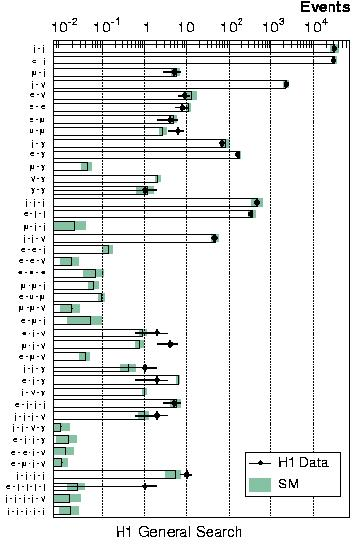# A general Search For New Phenomena in ep Scattering at HERA

High-energy electron-proton interactions provide a testing ground for the Standard Model of particle physics complementary to e+ e- and p pbar scattering. It is widely believed that the Standard Model is incomplete and many specific extensions to it have been constructed during the last decades. HERA data have been used to test some of these models of new processes by looking for the particular signatures that they predict. In this paper, we take a novel and different approach. Rather than assuming a particular extension to the Standard Model and searching for evidence for it, we look at the H1 data as a whole and perform a comprehensive and generic search for any deviations from the Standard Model prediction at large transverse momenta. Since no assumptions are made concerning the characteristics of any Standard Model extension, this approach might discover unexpected manifestations of new physics. It also allows us to answer interesting questions such as `How compatible are H1 data with the Standard Model overall?'

For the first time, all high PT final state configurations in an experiment, involving electrons, muons, jets, photons or neutrinos, are systematically investigated in a single analysis. The phase space region studied for these particles is PT > 20 GeV and 10o < theta < 140o. Events are classified into exclusive event classes according to the number and types of particles detected in the final state (e.g. electron-jet or muon-jet-neutrino).In a first analysis step, the total numbers of events in each class are compared with the Standard Model expectation, as shown in the figure. The most heavily populated classes are jet-jet and electron-jet, corresponding to dijet photoproduction and neutral-current DIS, respectively. There are around 30,000 events in each of these classes. At the other extreme, the analysis considers all classes where the Standard Model prediction is larger than 10-2 events, corresponding to cross sections as small as 0.1 fb for the 116 pb-1 studied. An impressive overall agreement with the predictions is obtained, illustrating the good understanding of Standard Model physics at HERA and of the H1 detector response to the different types of particle.

In a second analysis step, the distributions of the invariant mass and the scalar sum of transverse momenta of the particles are studied for each class. Since new physics may be visible as an excess or a deficit in some region of one of these distributions, they are systematically investigated using a dedicated statistical algorithm which locates the region with the largest deviation of the data from the Standard Model prediction. To quantify the seriousness of the disagreement, the probability of observing a deviation anywhere in the distribution which is at least as significant as that observed is calculated.

No highly significant deviations are observed in most of the event classes. In fact the only class which shows a deviation with a probability of less than 1% is that with a muon, a jet and a neutrino. The excess of events in this channel has also been previously reported by H1 (see e.g. here , here and here ). Considering only this one channel, the probability for the Standard Model to fluctuate to produce an excess at least as large as that observed is estimated to be about 1% from the invariant mass distribution and 0.1% from the transverse momentum distribution. However, the observation no longer looks quite so unlikely when it is considered that the excess could have occurred in any of the classes studied. The probability to observe a more significant deviation in any channel is estimated to be 3% (invariant mass distribution) and 28% (transverse momentum distribution).